• (矩阵的创建，numpy，应元素相乘）具有不错的参考意义，希望能够帮助到大家! 部分代码用图片方式呈现出来，方便各位观看与收藏！ 提示： python的numpy库提供矩阵运算的功能，因此我们在需要矩阵运算的时候，需要...
前言：
今天为大家带来的内容是，总结python的常见矩阵运算！(矩阵的创建，numpy，应元素相乘）具有不错的参考意义，希望能够帮助到大家!
部分代码用图片方式呈现出来，方便各位观看与收藏！
提示：
python的numpy库提供矩阵运算的功能，因此我们在需要矩阵运算的时候，需要导入numpy的包。
一.numpy的导入和使用
from numpy import *;#导入numpy的库函数
import numpy as np; #这个方式使用numpy的函数时，需要以np.开头。

二.矩阵的创建
由一维或二维数据创建矩阵
from numpy import *;
a1=array([1,2,3]);
a1=mat(a1);

创建常见的矩阵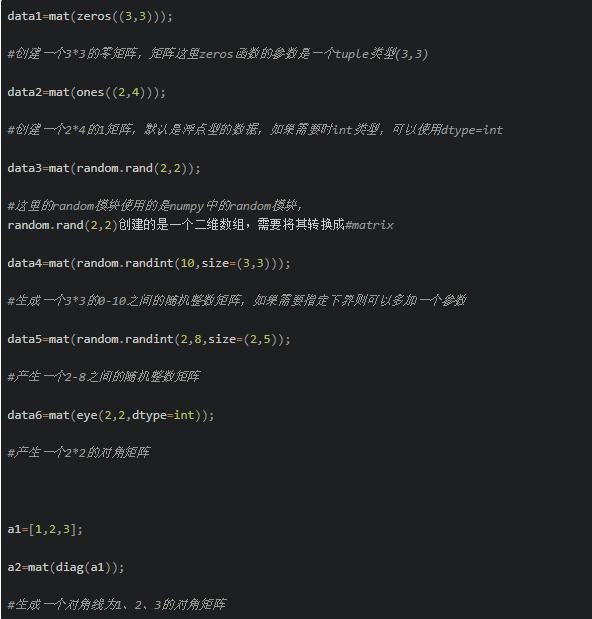三.常见的矩阵运算
1. 矩阵相乘
a1=mat([1,2]);
a2=mat([,]);
a3=a1*a2;
#1*2的矩阵乘以2*1的矩阵，得到1*1的矩阵

2. 矩阵点乘
矩阵对应元素相乘
a1=mat([1,1]);
a2=mat([2,2]);
a3=multiply(a1,a2);

矩阵点乘
a1=mat([2,2]);
a2=a1*2;

3.矩阵求逆，转置
矩阵求逆
a1=mat(eye(2,2)*0.5);
a2=a1.I;
#求矩阵matrix([[0.5,0],[0,0.5]])的逆矩阵

矩阵转置
a1=mat([[1,1],[0,0]]);
a2=a1.T;

4.计算矩阵对应行列的最大、最小值、和。
a1=mat([[1,1],[2,3],[4,2]]);

计算每一列、行的和
a2=a1.sum(axis=0);//列和，这里得到的是1*2的矩阵
a3=a1.sum(axis=1);//行和，这里得到的是3*1的矩阵
a4=sum(a1[1,:]);//计算第一行所有列的和，这里得到的是一个数值

计算最大、最小值和索引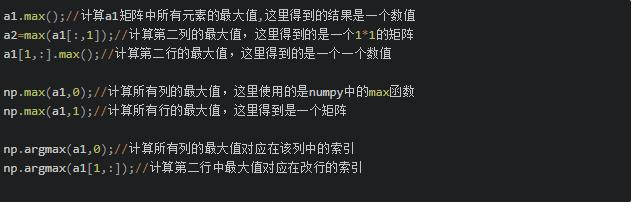5.矩阵的分隔和合并
矩阵的分隔，同列表和数组的分隔一致。
a=mat(ones((3,3)));
b=a[1:,1:];//分割出第二行以后的行和第二列以后的列的所有元素

矩阵的合并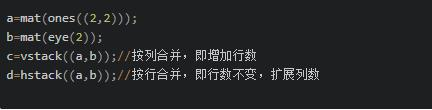四.矩阵、列表、数组的转换
列表可以修改，并且列表中元素可以使不同类型的数据，如下：
l1=[,'hello',3];

numpy中数组，同一个数组中所有元素必须为同一个类型，有几个常见的属性：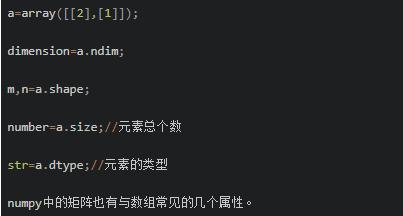它们之间的转换：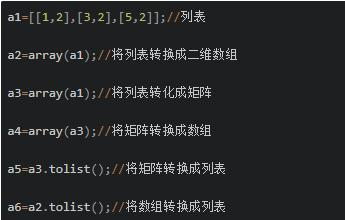这里可以发现三者之间的转换是非常简单的，这里需要注意的是，当列表是一维的时候，将它转换成数组和矩阵后，再通过tolist()转换成列表是不相同的，需要做一些小小的修改。如下：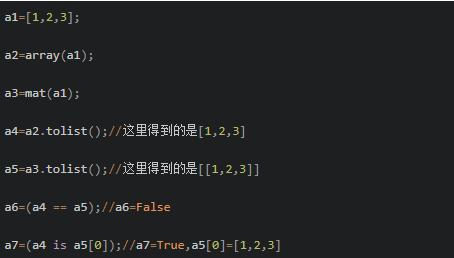矩阵转换成数值，存在以下一种情况：
dataMat=mat();
val=dataMat[0,0];//这个时候获取的就是矩阵的元素的数值，而不再是矩阵的类型

以上就是本文的全部内容，希望对大家的学习有所帮助
最后，小编想说一句话：我是一名python开发工程师，这里有我自己整理了一套最新的python系统学习教程，包括从基础的python脚本到web开发、爬虫、数据分析、数据可视化、机器学习等。想要这些资料的可以加群：877562786

展开全文python
• 当使用“*”的时候，如果两个矩阵之间的形状不能对应上，则会因为无法匹配而报错。当然如果只有行或者列对应不上可以通过广播使其行和列得到一一对应。 当使用“np.dot()”时，需要一个矩阵为(4,3)，另一个矩...
注意矩阵乘法运算中的“*”和“np.dot()”是不一样的。
“*”的意思是给定一个大小为(4,3)的矩阵A和一个大小为(4,3)的矩阵B，两者使用“A*B”得到的矩阵的形状还是(4,3)。
当使用“*”的时候，如果两个矩阵之间的形状不能对应上，则会因为无法匹配而报错。当然如果只有行或者列对应不上可以通过广播使其行和列得到一一对应。
当使用“np.dot()”时，需要一个矩阵为(4,3)，另一个矩阵为(3,4)，这样得到的矩阵的形状为(4,4)。
展开全文• 矩阵运算基础知识参考：矩阵的运算及其规则 1) matrix multiplication 矩阵乘法： (m,n) x (n,p) –&amp;amp;gt; (m,p) # 矩阵乘法运算前提：矩阵1的列=矩阵2的行 3种用法： np.dot(matrix_a, ...
矩阵运算基础知识参考：矩阵的运算及其规则

注意区分数组和矩阵的乘法运算表示方法（详见第三点代码）
1) matrix multiplication
矩阵乘法： (m,n) x (n,p) --> (m,p) # 矩阵乘法运算前提：矩阵1的列=矩阵2的行 3种用法： np.dot(matrix_a, matrix_b) == matrix_a @ matrix_b == matrix_a * matrix_b
2） element-wise product ： 矩阵对应元素相乘
1种用法：np.multiply(matrix_c, matrix_d) 对于nd.array()类型而言，数组 arrA * arrB 只能element-wise produt(对应元素相乘)
# -*- coding: utf-8 -*-
"""
Created on Thu Jul 26 14:22:40 2018

"""

import numpy as np

a = np.array([[1,2],[3,4],[11,12]])
b = np.array([[5,6,13],[7,8,14]])
c = np.array([[1,2,13],[3,4,25],[11,12,23]])
d = np.array([[5,6,2],[7,8,29],[13,14,15]])

matrix_a = np.matrix(a)         # (3,2)
matrix_b = np.matrix(b)         # (2,3)
matrix_c = np.matrix(c)         # (3,3)
matrix_d = np.matrix(d)         # (3,3)

print(type(a),type(matrix_a))  # <class 'numpy.ndarray'> <class 'numpy.matrixlib.defmatrix.matrix'>
mat_a = np.mat(a)
print(type(a),type(matrix_a))  # <class 'numpy.ndarray'> <class 'numpy.matrixlib.defmatrix.matrix'>

'''
# 1) matrix multiplication
矩阵乘法： (m,n) x (n,p) --> (m,p)    # 矩阵乘法运算前提：矩阵1的列=矩阵2的行
3种用法： np.dot(matrix_a, matrix_b) == matrix_a @ matrix_b == matrix_a * matrix_b
'''
method_1 = matrix_a @ matrix_b
method_2 = np.dot(matrix_a, matrix_b)

print(method_1)
#[[ 19  22  41]
# [ 43  50  95]
# [139 162 311]]
print(method_2 == method_1)
#[[ True  True  True]
# [ True  True  True]
# [ True  True  True]]
print(matrix_c * matrix_d == matrix_c @ matrix_d)
#[[ True  True  True]
# [ True  True  True]
# [ True  True  True]]

'''
# 2） element-wise product ： 矩阵对应元素相乘
1种用法：np.multiply(matrix_c, matrix_d)
对于nd.array()类型而言，数组 arrA * arrB 只能element-wise produt(对应元素相乘)
'''
print(matrix_c, matrix_d, sep='\n')
#[[ 1  2 13]
# [ 3  4 25]
# [11 12 23]]
#[[ 5  6  2]
# [ 7  8 29]
# [13 14 15]]

method_1 = np.multiply(matrix_c, matrix_d)      # 对应位置元素相乘

print(method_1)
#[[  5  12  26]
# [ 21  32 725]
# [143 168 345]]

3) 矩阵乘法和数组乘法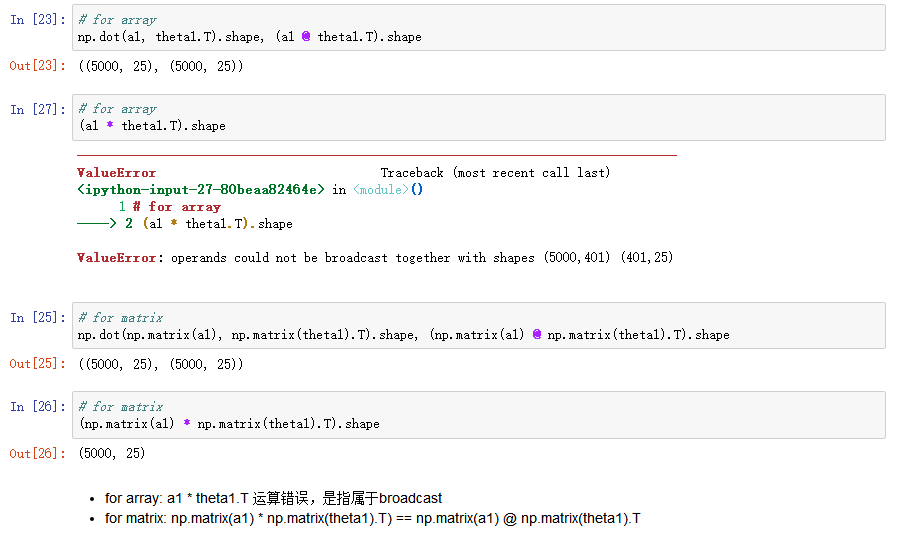展开全文• 把向量或矩阵中所有元素相乘 import numpy as np mat= np.array([[1, 2, 3], [1, 2, 3], [1, 2, 3]]) print(np.prod(mat)) 结果： 216 示例2. 指定 axis Axis or axes along which a product is performed. 示例...
numpy.prod(a, axis=None, dtype=None, out=None, keepdims=, initial=, where=)
Return the product of array elements over a given axis.
官方链接
a [array_like] Input data.
axis [None or int or tuple of ints, optional] Axis or axes along which a product is performed. The default, axis=None, will calculate the product of all the elements in the input array. If axis is negative it counts from the last to the first axis.
dtype [dtype, optional] The type of the returned array, as well as of the accumulator in which the elements are multiplied. The dtype of a is used by default unless a has an integer dtype of less precision than the default platform integer. In that case, if a is signed then the platform integer is used while if a is unsigned then an unsigned integer of the same precision as the platform integer is used.
out [ndarray, optional] Alternative output array in which to place the result. It must have the same shape as the expected output, but the type of the output values will be cast if necessary.
keepdims [bool, optional] If this is set to True, the axes which are reduced are left in the result as dimensions with size one. With this option, the result will broadcast correctly against the input array.
If the default value is passed, then keepdims will not be passed through to the prod method of sub-classes of ndarray, however any non-default value will be. If the sub-class’ method does not implement keepdims any exceptions will be raised.
initial [scalar, optional] The starting value for this product. See reduce for details.
where [array_like of bool, optional] Elements to include in the product. See reduce for details.
若指定了axis，把数组a沿着维度axis进行切片，并把切片后得到的一系列数组每个对应位置的元素相乘(element-wise product)，如果axis未指定，则对数组进行扁平化后计算所有元素的乘积.
示例1. 把向量或矩阵中所有元素相乘
import numpy as np
mat= np.array([[1, 2, 3], [1, 2, 3], [1, 2, 3]])
print(np.prod(mat))

结果：
216

示例2. 指定 axis，实现某一维度的元素相乘
例如对于二维数组，指定axis=0，则按照行进行切片，然后对应位置相乘(element-wise product)，得到的是每一列元素相乘的乘积。 相当于np.multiply.reduce(mat,axis=0)
import numpy as np
mat= np.array([[1, 2, 3], [1, 2, 3], [1, 2, 3]])
print(np.prod(mat,axis=0))
print(np.multiply.reduce(mat,axis=0))

结果：
[ 1  8 27]
[ 1  8 27]

示例3. 指定where，实现只对某些index的元素进行相乘
如果要计算 [1,2,3,4,5,6] 中index为 [0,2,4] 三个元素的乘积
np.prod([1., np.nan, 3.], where=[True, False, True])

结果
3.0

展开全文python 机器学习 数据挖掘 深度学习python
• import numpy as np a = [[0,0], [3,4]] b = [[1,2], [1,2]] a = np.array(a) b = np.array(b) # 矩阵乘法 ...# 对应相乘 res3 = np.multiply(a,b) res4 = a*b print(res3) print(res4) ...线性代数 python 机器学习
• 对应元素相乘 element-wise product: np.multiply(), 或 * y1 = np.array([0, 0, 0, 1, 1, 1, 2, 2, 2]) y2 = np.array([0, 0, 0, 1, 1, 1, 2, 2, 2]) y1*y2 Out: array([0, 0, 0, 1, 1, 1, 4, 4, 4]) np....python 点乘 转置
• 在机器学习中，会遇见两个...也叫向量的内积、数量积，对两个向量执行点乘运算，就是对这两个向量对应位一一相乘之后求和的操作，点乘的结果是一个标量。 1、设n1是3 x 3 的矩阵，n2 是1 x 3 的矩阵 如上图所示...
• A*B是矩阵（向量）对应元素相乘 np.dot(A,B)是矩阵乘法 A,B形式相同，各个元素相乘=B*A A,B满足矩阵相乘的条件。 A为2x2，B为1x2，所以是A的每一行和B各元素对应相乘 A为2x2，B为2x1，所以是A的每...python
• 对应相乘（简称点乘） np.multiply(A, B) 或者 A * B A.mul(B) 矩阵相乘、两种积（内积、点积） np.dot(A, B) A.mm(B) 1. numpy(ndarray) A = np.array([[1, 2], [-...
• 看到numpy中array的乘法运算时，由于array的乘法符号表示与常见的线性代数中矩阵运算符号恰好相反，脑子总是绕不过弯。为了更好的记忆和使用array的乘法符号，现记录一下自己在查阅资料后关于numpy中array乘法运算...python
• 1. 问题ndarray 是 NumPy 的基础元素NumPy 又主要是用来进行矩阵运算的。那么具体来说，ndarray 是如何进行普通矩阵运算的呢？2. 分析首先，在矩阵用 +-*/ 这些常规操作符操作的时候，是对元素进行操作。这和其他...
• 1. 当为array的时候，默认d*f就是对应元素的乘积，multiply也是对应元素的乘积，dot（d,f）会转化为矩阵的乘积， dot点乘意味着相加，而multiply只是对应元素相乘，不相加 2. 当为...
• numpy数组不同维度相乘 ...两个形状相同的矩阵做 * ,其实就是对应元素相乘 x = np.array([1,2,3]) print(x.shape) print(x) y = np.array([1,2,3,4,5,6]) z = y[:, None] print(z) print(x*z) x = np....
• import numpy as np ...#两个数组上对应位置相乘，有两种方法 a=np.multiply(X,Y) #这种方法也适用于两个计算对象是列表，如X1=[[2,4,5],[5,3,6]]，Y1=[[4,6,3],[4,6,3]] b=X*Y print('a=','\n',a,'.python 线性代数
• 1. 当为array的时候，默认d*f就是对应元素的乘积，multiply也是对应元素的乘积，dot（d,f）会转化为矩阵的乘积， dot点乘意味着相加，而multiply只是对应元素相乘，不相加 2. 当为mat的时候，默认d...数组
• #1、求m,n中矩阵对应元素的和，元素的乘积 m = [[1,2,3], [4,5,6], [7,8,9]] n = [[2,2,2], [3,3,3],[4,4,4]] #m,n中矩阵对应元素的和 m = [[1,2,3], [4,5,6], [7,8,9]] n = [[2,2,2], [3,3,3],[4,4,4]] m ...python
• <code class="language-python">import numpy as np a = np.array([1, 2]) b =...而且感觉是第一个矩阵的行向量依次与第二个数组对应元素相乘,返回了一个数组,可以解释一下其中的原理吗?</p>python 有问必答
• Python中的几种矩阵乘法 1. 同线性代数中矩阵乘法的定义： np.dot() np.dot(A, B)：对于二维矩阵，计算真正意义上的矩阵乘积...import numpy as np # 2-D array: 2 x 3 two_dim_matrix_one = np.array([[1, 2, 3]...
• 矩阵乘法，又称矩阵内积、点积， 在Numpy中的实现方法： ...Hadamard乘积，将矩阵中相同位置的元素相乘，又称element-wise product， 在Numpy中的实现方法： 1，‘*’运算符，最简单 2， np.multiply()函数，还行 ...
• 创建NumPy矩阵 NumPy对于多维数组的运算，默认情况下并不进行矩阵运算。如果需要对数组进行矩阵运算，则可以调用相应的函数。 在NumPy中，矩阵是ndarray的子类。 在NumPy中，数组和矩阵有着重要的区别。NumPy提供了...计算 属性
• 1、当为array的时候，默认d*f就是对应元素的乘积，multiply也是对应元素的乘积，dot（d,f）会转化为矩阵的乘积， dot点乘意味着相加，而multiply只是对应元素相乘，不相加 2、当为mat的时候，默认d*f就是矩阵的乘积...
• 分类: 元素相乘:multply() 矩阵相乘:d...
• numpy中matrix 和 array的区别Numpy matrices必须是2维的,但是 numpy arrays (ndarrays) 可以是多维的（1D，2D，3D····ND）. Matrix是Array的一个小的分支，包含于Array。所以matrix 拥有array的所有特性。python matrix array
• (矩阵的创建，numpy，应元素相乘)具有不错的参考意义，希望能够帮助到大家!部分代码用图片方式呈现出来，方便各位观看与收藏！提示：python的numpy库提供矩阵运算的功能，因此我们在需要矩阵运算的时候，需要导入...
• 分类: 元素相乘:multply() 矩阵相乘:dot()、matmul()、’@’ ’ * ': 是特别的。在数组操作中，作为元素相乘；在矩阵操作中作为矩阵相乘。 以下举例说明 import numpy as np ...print(a1 * b1) # 对应元素相乘...
• numpy矩阵可以由矩阵，数组甚至列表转换而来，接下来看一下实例 import numpy as np # 创建矩阵 ---必须是二维的 # mat 生成矩阵的方法1 m1 = np.mat("1 2 3;4 5 6;7 8 9") m2 = np.mat([[1,2,3],[4,5,6],[7,8,9...
• import numpy as np # 可以使用np.mat np.asmatrix np.bmat 来创建矩阵 # np.mat 和 np.asmatrix 是相同 # 可以将特殊字符串转化为矩阵 m1 = np.mat('1 2 3;4 5 6;7 8 9') print('m1:\n', m1) print('m1 类型：\n',...数据分析 python...